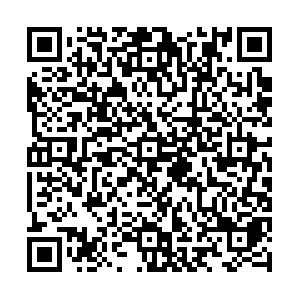# The effect of hadronic scatterings on the measurement of vector meson spin alignments in heavy-ion collisions

• Spin alignments of vector mesons and hyperons in relativistic heavy-ion collisions have been proposed as signals of global polarization. The STAR experiment first observed the $\Lambda$polarization. Recently, the ALICE collaboration measured the transverse momentum ($p_T$) and the collision centrality dependence of $K^*$, and $\phi$spin alignments during Pb-Pb collisions at $\sqrt {{s_{{\rm NN}}}}$= 2.76 TeV. A large signal is observed in the low $p_T$region of mid-central collisions for $K^*$, while the signal is much smaller for $\phi$, and these have not been understood yet. Since vector mesons have different lifetimes and their decay products have different scattering cross sections, they suffer from different hadronic effects. In this paper, we study the effect of hadronic interactions on the spin alignment of $K^*$, $\phi$, and $\rho$mesons in relativistic heavy-ion collisions with a multi-phase transport model. We find that hadronic scatterings lead to a deviation of the observed spin alignment matrix element $\rho_{00}$away from the true value for $\rho$and $K^*$mesons (with a bigger effect on $\rho$) while the effect is negligible for the $\phi$meson. The effect depends on the kinematic acceptance: the observed $\rho_{00}$value is lower than the true value when the pseudorapidity ($\eta$) coverage is small, while there is little effect when the $\eta$coverage is large. Hence, this study provides valuable information to understand the vector meson spin alignment signals observed during the experiments.
••Get Citation
Diyu Shen, Jinhui Chen and Zi-Wei Lin. The effect of hadronic scatterings on the measurement of vector meson spin alignments in heavy-ion collisions[J]. Chinese Physics C. doi: 10.1088/1674-1137/abe763
Diyu Shen, Jinhui Chen and Zi-Wei Lin. The effect of hadronic scatterings on the measurement of vector meson spin alignments in heavy-ion collisions[J]. Chinese Physics C.Milestone
Received: 2021-01-10
Article Metric

Article Views(150)
PDF Downloads(14)
Cited by(0)
Policy on re-use
To reuse of subscription content published by CPC, the users need to request permission from CPC, unless the content was published under an Open Access license which automatically permits that type of reuse.
###### 通讯作者: 陈斌, bchen63@163.com
• 1.

沈阳化工大学材料科学与工程学院 沈阳 110142

Title:
Email:

## The effect of hadronic scatterings on the measurement of vector meson spin alignments in heavy-ion collisions

• 1. Shanghai Institute of Applied Physics, Chinese Academy of Sciences, Shanghai 201800, China
• 2. University of Chinese Academy of Sciences, Beijing 100049, China
• 3. Institute of Modern Physics and Key Laboratory of Nuclear Physics and Ion-beam Application (MOE), Fudan University, Shanghai 200433, China
• 4. Key Laboratory of Quarks and Lepton Physics (MOE) and Institute of Particle Physics, Central China Normal University, Wuhan 430079, China
• 5. Department of Physics, East Carolina University, Greenville, North Carolina 27858, USA

Abstract: Spin alignments of vector mesons and hyperons in relativistic heavy-ion collisions have been proposed as signals of global polarization. The STAR experiment first observed the $\Lambda$polarization. Recently, the ALICE collaboration measured the transverse momentum ($p_T$) and the collision centrality dependence of $K^*$, and $\phi$spin alignments during Pb-Pb collisions at $\sqrt {{s_{{\rm NN}}}}$= 2.76 TeV. A large signal is observed in the low $p_T$region of mid-central collisions for $K^*$, while the signal is much smaller for $\phi$, and these have not been understood yet. Since vector mesons have different lifetimes and their decay products have different scattering cross sections, they suffer from different hadronic effects. In this paper, we study the effect of hadronic interactions on the spin alignment of $K^*$, $\phi$, and $\rho$mesons in relativistic heavy-ion collisions with a multi-phase transport model. We find that hadronic scatterings lead to a deviation of the observed spin alignment matrix element $\rho_{00}$away from the true value for $\rho$and $K^*$mesons (with a bigger effect on $\rho$) while the effect is negligible for the $\phi$meson. The effect depends on the kinematic acceptance: the observed $\rho_{00}$value is lower than the true value when the pseudorapidity ($\eta$) coverage is small, while there is little effect when the $\eta$coverage is large. Hence, this study provides valuable information to understand the vector meson spin alignment signals observed during the experiments.

### HTMLI.   INTRODUCTIONII.   MODEL AND METHODOLOGYIII.   RESULTS AND DISCUSSION
Reference (40)
PDF查看关注分享

Top

### 目录/DownLoad:  Full-Size Img  PowerPoint